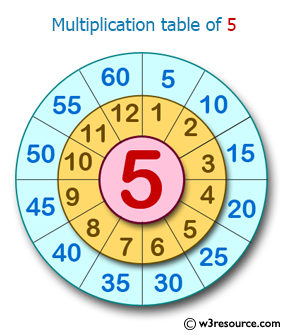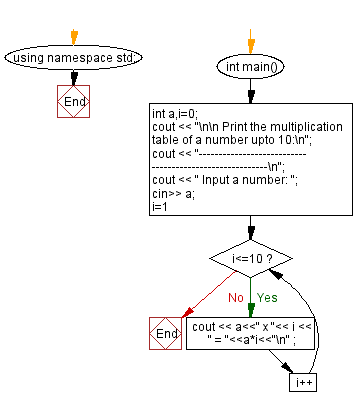﻿ C++ : Takes a number and prints multiplication table upto 10

# C++ Exercises: Takes a number as input and prints its multiplication table upto 10

## C++ Basic: Exercise-38 with Solution

Write a C++ program that takes a number as input and prints its multiplication table up to 10.

Pictorial Presentation:Sample Solution:

C++ Code :

``````#include <iostream>
using namespace std;

int main()
{
int a,i=0;
cout << "\n\n Print the multiplication table of a number upto 10:\n";
cout << "--------------------------------------------------------\n";
cout << " Input a number: ";
cin>> a;
for (i=1;i<=10;i++)
{
cout << a<<" x "<< i << " = "<<a*i<<"\n" ;
}

}
``````

Sample Output:

``` Print the multiplication table of a number upto 10:
--------------------------------------------------------
Input a number: 5
5 x 1 = 5
5 x 2 = 10
5 x 3 = 15
5 x 4 = 20
5 x 5 = 25
5 x 6 = 30
5 x 7 = 35
5 x 8 = 40
5 x 9 = 45
5 x 10 = 50
```

Flowchart:C++ Code Editor:

What is the difficulty level of this exercise?

﻿

## C++ Programming: Tips of the Day

Why is there no std::stou?

The most pat answer would be that the C library has no corresponding "strtou", and the C++11 string functions are all just thinly veiled wrappers around the C library functions: The std::sto* functions mirror strto*, and the std::to_string functions use sprintf.

Ref: https://bit.ly/3wtz2qA

We are closing our Disqus commenting system for some maintenanace issues. You may write to us at reach[at]yahoo[dot]com or visit us at Facebook